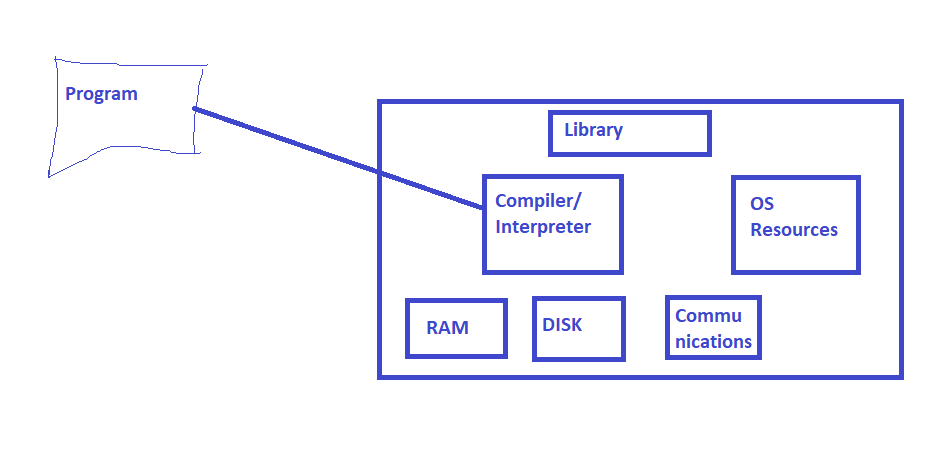## Basic Program Components (Internal)## Program Conversions into Machine Language

1. Compiler
2. Interpreter
3. Hybrid: Compiler + Interpreter

## In which order does Programs Execute your code.

• Any program executes your code sequentially (statement by statement)

``````f1 = 10
f2 = 20
result = f1+f2
print(result)
``````
• If you execute this program execution happens sequentially.
• Manually executing Programs is called as debugging.

### Scenarios

#### print all the even numbers below 1000

• Note:

• Datatypes: integer and boolean
• Operators: + – * / % = == < >
• Solution 1

``````integer index = 1
integer max = 1000
repeat till index < 1000
is index%2 == 0
print index
index = index + 1
``````
• Solution 2
``````integer index = 1
integer max = 1000
integer start = 2
repeat till start * index < max
print start * index
index = index+1
``````
• Convert into Python
• Find the equivalent operators
• Find the equivalent data types
• Find the python syntax

## Conditional Statements

• Skipping some statements when conditions are (not) met
• IF Statement
``````IF boolean expression THEN
block begin
statement 1
..
..
Statement n
block end
``````
• IF ELSE
``````IF boolean expression THEN
true_block begin
statement 1
..
..
Statement n
true_block end
ELSE
false_block begin
statement 1
..
..
Statement n
false_block end

``````
• CASE/Switch
`````` CASE(Expression):
case A:
A_block begin
...
...
A_block end
...
...
...
default:
default block begin
...
...
default block end
``````

## Looping Statement

• Repeating some line when conditions are (not) met
• WHILE
``````WHILE (Boolean expression)
TRUE-Block Begins
Statement 1
..
..
Statement n
TRUE Block Ends
``````
• FOR
• FOR EACH

### Python Environment Setup

1. Install Visual Studio Code from here
2. Install python from here

This site uses Akismet to reduce spam. Learn how your comment data is processed.